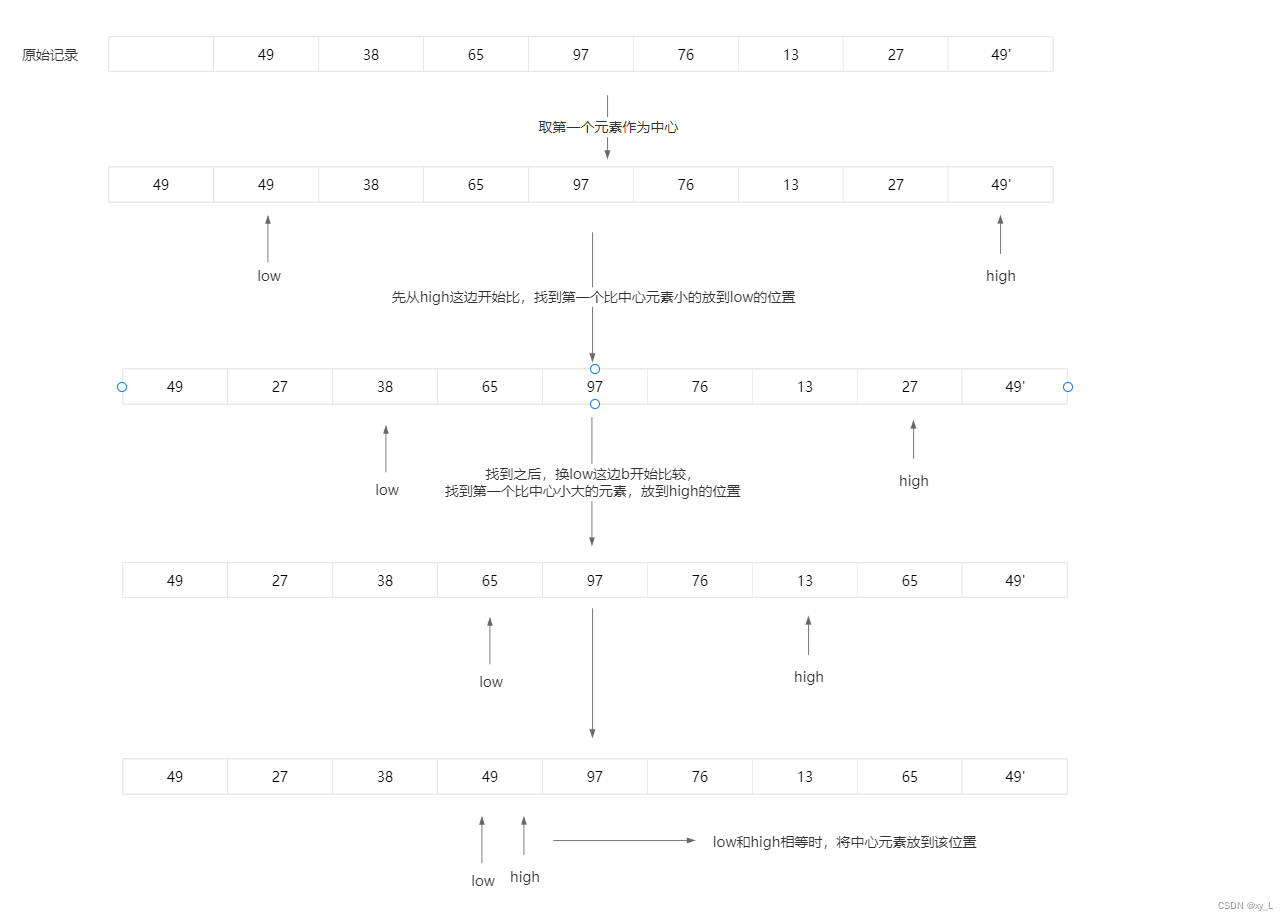# 8.3 数据结构——交换排序

## 8.3.1 冒泡排序

``````void Bubble_Sort(SQList *L)
{
RedType x; //交换时临时存储
for (int i = 1; i < L->length; i++)
for (int j = 1; j <= L->length - i; j++)
{
if (L->r[j].key > L->r[j+1].key)
{
x = L->r[j];
L->r[j] = L->r[j+1];
L->r[j+1] = x;
}
}
}``````

``````void Bubble_Sort(SQList *L)
{
int flag = 1;
RedType x;
for (int i = 1; i < L->length; i++)
{
flag = 0;
for (int j = 1; j <= L->length-i; j++)
{
if (L->r[j].key > L->r[j+1].key)
{
flag = 1;
x = L->r[j];
L->r[j] = L->r[j+1];
L->r[j+1] = x;
}
}
}
}``````

## 8.3.2 快速排序

（1）任取一个元素（如：第一个）为中心；

（2）所有比它小的元素一律前放，比它大的元素一律后放，形成左右两个子表；

（3）对各子表重新选择中心元素，并依此规律调整，直到每个子表的元素只剩一个。这一趟之后就形成了两个子表，再以相同的方法继续对左右子表排序。

``````void Quick_Sort(QSList *L, int low, int high)
{
if (low < high)
{
int pivotloc = Partition(L, low, high);
Quick_Sort(L, low, pivotloc-1);
Quick_Sort(L, pivotloc+1, high);
}
}

int Partition(SQList *L, int low, int high)
{
L->r = L->r[low];
KeyType pivotkey = L->r[low].key;
while (low < high)
{
while (low < high && L->r[high].key >= pivotkey)
high--;
L->[low] = L->r[high];
while (low < high && L->r[low] <= pivotkey)
low++;
L->r[high] = L->r[low];
}
L->r[low] = L->r;
return low;
}``````